Trial ends in
JoVE Science Education
Structural Engineering

A subscription to JoVE is required to view this content.
You will only be able to see the first 20 seconds.

Dynamics of Structures
Science Education (Structural Engineering)
Stress-Strain Characteristics of Aluminum
Science Education (Structural Engineering)
Tests of Hardened Concrete in Tension

Dynamics of Structures

Overview

Source: Roberto Leon, Department of Civil and Environmental Engineering, Virginia Tech, Blacksburg, VA

It is rare nowadays that a whole year goes by without a major earthquake event wreaking havoc somewhere around the world. In some cases, like the 2005 Banda Ache earthquake in Indonesia, the damage involved large geographic areas and casualties in the six figures. In general, the number and intensity of earthquakes is not increasing, however, the vulnerability of the built environment is rising. With increasing unregulated urbanization around seismically active areas, such as the Circum-Pacific "belt of fire," sea rising in low-laying coastal area, and increasing concentrations of both energy production/distribution and digital/telecommunication network critical nodes in vulnerable areas, it is clear that earthquake-resistant design is key to future community resilience.

Designing structures to resist earthquake damage has progressed greatly in the last 50 years, primarily through work in Japan following the 1964 Niigata Earthquake, and in the United States following the 1971 San Fernando Valley Earthquake. The work has advanced along three parallel tracks: (a) experimental work aimed at developing improved construction techniques to minimize damage and loss of life; (b) analytical studies based on advanced geometrical and non-linear material models; and, (c) synthesis of the results in (a) and (b) into design code provisions that improve the ability of structures to resist unexpected loads.

Seismic testing in a laboratory setting is often difficult and expensive. Testing is primarily carried out using the following three techniques:

1. Quasi-static testing (QST), where parts of a structure are tested using slowly applied and equivalently predetermined lateral deformations with idealized boundary conditions. This technique is particularly useful to assess the effects of structural detailing on the toughness and deformation capacity of particular parts of structures.
2. Pseudo-dynamic testing (PSDT), where loads are also applied slowly, but the dynamic effects are taken into account by solving the equations of motion as the test progresses and by utilizing direct test feedbacks (primarily the instantaneous stiffness) to assess the actual stiffness and damping characteristics of the structure.
3. Shake tables, where scale models of complete structures are subjected to input motions using a hydraulically actuated base or foundation. Shake tables represent a more faithful testing technique, as the structure is not artificially restrained, the input is true ground motion, and the resulting forces are truly inertial ones, as one would expect in a real earthquake. However, the power requirements are enormous, and only a few shake tables capable of working at nearly full-scale exist around the world. Globally, there is only one large shake table capable of carrying out tests on full-scale structures, which is the shake table at the E-Defense facility in Japan, built in the aftermath of the 1985 Kobe earthquake.

In this experiment, we will utilize a small shake table and model structures to study the dynamic behavior characteristics of some structural models. It is these dynamic characteristics, principally the natural frequency and damping, as well as the quality of the structural detailing and construction, which make structures more or less vulnerable to earthquakes.

Principles

There is a fundamental difference between the usual gravity (self-weight) loads that act on a structure, which are quasi-static (i.e., they change very slowly, or not at all with time), and those produced by hurricanes, blasts, and earthquakes, which are extremely dynamic in nature. In the case of hurricanes and other wind loads, it is possible to model their effects as equivalent static pressures in the laboratory as the frequency of the winds is very long compared to the fundamental natural frequency of the typical structure. Important exceptions to this include flexible structures, such as long-span cable-stayed and suspension bridges, tall masts, and wind turbine structures, where the natural frequency of the structure can match that of the wind gusts or straight winds. In the case of earthquakes, the loads are primarily inertial as the ground moves, and the structure tends to stay still. In this case, the loading depends on the actual mass, stiffness, and damping of the structure, and the quantities of interest are the accelerations, velocities, and displacements around the structure. This second set of quantities is very difficult to reproduce accurately in the laboratory if shake tables are not available.

Using basic physics, like Newton's Second Law, one can simplify the problem of the equilibrium of a structure (such as a bridge or a frame with rigid beam), which is subject to ground motions (ug), to that of a single degree-of-freedom mass (m) with stiffness (k) and damping (c) characteristics. The latter two can be represented by a spring in which the force is proportional to the displacement (u) as well as a dashpot in which the forces are proportional to the velocity (v) (Figure 1). These components can be combined in parallel and/or series to model different structural configurations.

Stiffness is defined as the force required to deform the structure by a unit amount. Suppose that one loads a cantilever beam with a known force (P) and measure its elastic deformation at the tip (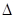). The stiffness is defined as k = P/. For the simple elastic cantilever system shown, k= L3/3EI, where L is the length of the cantilever, I is its moment of inertia, and E is Young's modulus for the material used. Next, imagine what happens if one removes the force suddenly, thereby allowing the cantilever to vibrate. One intuitively will expect the amplitude of vibrations to begin to decrease with every cycle. This phenomenon is called damping and refers to a series of complex internal mechanisms, such as friction, that tend to reduce the oscillations. The quantification of damping is described later in this lab, but it is important to note that at this point, not much is known about these mechanisms from either a theoretical or practical standpoint. A useful concept is to visualize the critical damping coefficient (ccr), which corresponds to the case where the cantilever will come to rest after just one complete oscillation.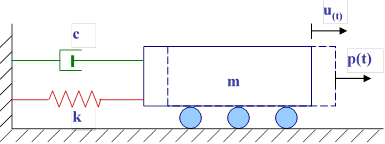Figure 1: Single degree of freedom system model.

Writing an equation of horizontal equilibrium of forces for the system pictured in Figure 1 leads to: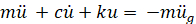(Eq. 1)

If we look at a simpler case for a moment, where we can ignore damping because its effects are negligible, and there is no external forcing function, Equation 1 becomes the linear homogeneous second-order differential equation: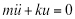(Eq. 2)

whose solution is of the form: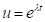(Eq. 3)

Differentiating twice will give us: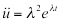(Eq. 4)

Substituting Equation 4 into Equation 2, yields: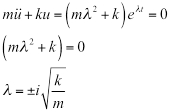(Eq. 5)

The general solution is: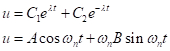(Eq. 6)

Where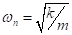is the undamped natural frequency of the system.

If this system is given an initial displacement (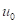) and/or an initial velocity (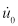), Equation 6 becomes: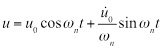(Eq. 7)

If we add the effect of damping (c) and define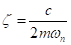, the damped natural frequency of the system becomes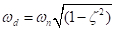and the equivalent to Equation 7 is: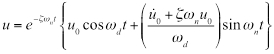(Eq. 8)

For the case of an initial displacement u0, Figure 2 shows the behavior for several values of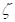.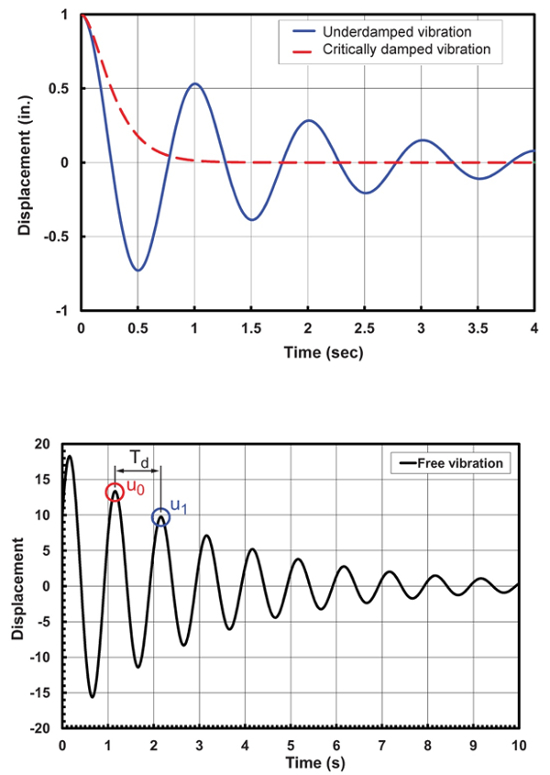Figure 2: Effect of damping on free vibrations: definition of critical damping (upper); calculation of damping from logarithmic decrement (lower).

If in Figure 2, one defines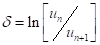, where un and un+1 are the displacement in successive cycles, then: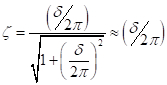(Eq. 9)

Going back to Equation 1, if the ground motion is taken as the sinusoidal function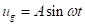, the analogue of Equation 8 is: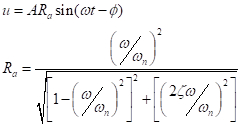(Eq. 10)

Where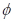is the phase lag, and Ra is the amplification response factor, whose plots are illustrated in Figure 3. Figure 3 shows that for low values of damping (<0.2), as the frequency of the forcing function approaches the natural frequency of the system, the response of the system becomes unstable, a phenomenon that is commonly referred to as resonance.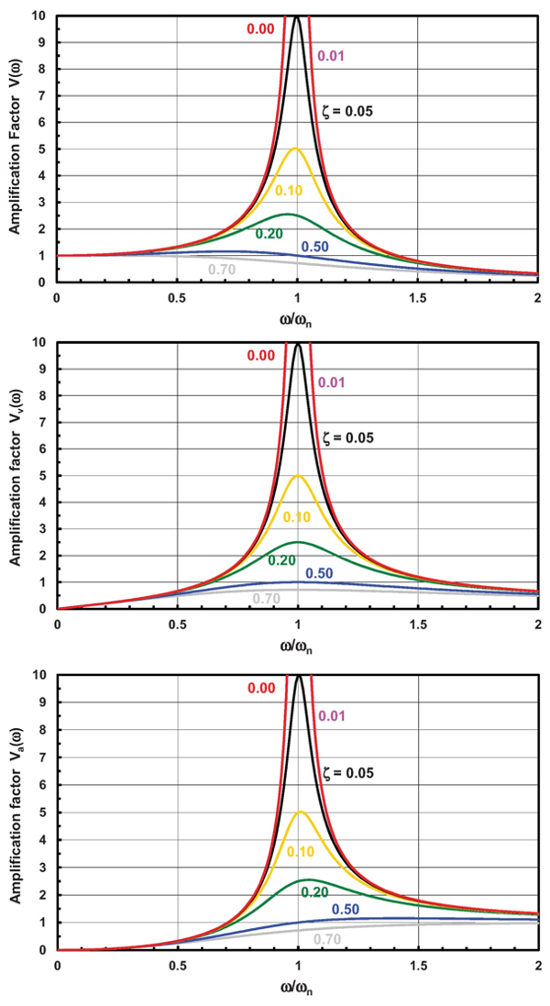Figure 3: Displacement, velocity and acceleration response.

In this lab, we will experimentally investigate the concepts and derivations behind Equations 1- 10 in the context of the dynamics of structures using a shake table.

Procedure

1. Models

1. First construct several structures using very thin, strong, rectangular, T6011 aluminum beams, 1/32 in. in width and having different lengths. To build the first model, insert one single cantilever with length of 12 in. to a very rigid wood block. Place a mass of 0.25 lb. to the tip of the cantilever.
2. Similarly, build other model structures by attaching cantilevers with different lengths to the same rigid wood block. Attach a 0.25 lbs. mass to the tip of each cantilever.
3. Prepare two other specimens simulating simple frame structures with flexible columns and rigid floors. These can be built of thin steel plates and rigid acrylic floor diaphragms. One structure will be a one-story and the other will be two stories. The floor diaphragms will be instrumented with accelerometers.

2. Apparatus

For these demonstrations a small, table top, electrically actuated, single degree of freedom shake table will be used. The apparatus consists basically of a small metal table riding on two guiding rails that is displaced by an electric motor. The displacement is digitally controlled by a computer that can input periodic (sine waves) or random accelerations (preprogrammed earthquake ground acceleration time histories). All control is through proprietary software or MatLab and Si mulLink type software. The input forcing function can be checked by comparing it to the output of an accelerometer attached to the table.

3. Procedure

1. Carefully mount the model with various cantilevers to the shake table, using bolts attached to the model's base. Turn on the shake table and using the software, slowly increase the frequency until the maximum response of the structure is obtained for each cantilever. Note that each cantilever enters resonance at a particular frequency. Record in a notebook the value of this frequency. Continue increasing the frequency until the displacements of all cantilevers reduce significantly.
2. Mount the one-story model structure to the shake table and repeat the procedure. Slowly sweep through frequencies until resonance is reached. Reset the software to run a typical ground acceleration time history (1940 El Centro) to show the random motions that occur during an earthquake.
3. Mount the two-story structure to the shake table and repeat the procedure. Note that two natural frequencies occur in this case.

Structural dynamics, or the analysis of structure's behavior when subjected to dynamic forces, is critical both for designing buildings able to resist earthquake and fatigue loads, and for providing occupant comfort in structures subjected to wind and other types of cyclic loads.

In order to develop resilient design strategies for our cities' infrastructures, we need to understand both the input, for example, the ground motion during seismic activity, and the output, or the structural response of the buildings. This issue can only be addressed through a combined analytical and experimental approach.

Seismic testing in a laboratory setting is carried out using shake tables, where scale models of complete structures are subjected to input motions using an electrically or hydraulically actuated base. This method represents a more faithful testing technique, as the structure is not artificially restrained, and the input is true ground motion.

This video will illustrate the principles of dynamic analysis by using a shake table and model structures to study the dynamic behavior characteristics of different structural models.

The usual self weight loads acting on a structure are quasi static because they change very slowly or not at all with time. In contrast, loads produced by hurricanes and blasts, for example, are extremely dynamic in nature.

During an earthquake, the ground moves with certain acceleration while the structure tends to stay still. As a consequence, the dynamic loads acting on a structure are inertial, and they depend on the mass, stiffness, and damping of the structure. To solve this problem analytically, we employ basic physics laws and simplified models of the actual structures.

For example, both a bridge and a frame with rigid beam can be simplified to a single degree of freedom system, consisting of an elastic cantilever with length L and mass m, stiffness k, and damping c. Alternatively, another model system can be represented by a mass attached to a spring of elastic constant k, as well as a dash pot with a damping coefficient c. These components can be combined in parallel and in series to model different structural configurations.

For our mass and spring model system, if the ground is moving the external force acting on this system is proportional with the ground acceleration. The other forces in the system are the elastic force in the spring, proportional to the displacement, as well as the reaction force in the dash pot, proportional to the velocity.

Using Newton's Second Law, we can write the equation of horizontal equilibrium of forces for this system. In the absence of external forces, and assuming the damping effects as negligible, this simplified equation has the following solution:

Here, wn is the undamped natural frequency of the system, and u0 is the initial displacement. If we add the effect of damping, the solution of the equation of motion is the following. Here the damped natural frequency of the system is expressed using the natural frequency and the damping coefficient.

The effective damping on the free oscillations of the system results in the decrease of the amplitude of vibrations with every cycle. Considering the displacements in two successive cycles, we can use the logarithmic decrement delta to calculate the damping constant zeta.

If the ground motion is taken as sinusoidal function, the solution for the equation of motion is given by the following function. Here phi is the phase lag, and R is the amplification response factor.

Let's plot this factor versus frequency ratio for different values of the damping coefficient zeta. For low values of damping, as the frequency of the forcing function approaches the natural frequency of the system, the response of the system becomes unstable, a phenomenon that is commonly referred to as resonance.

Now that you understand the theoretical concepts regarding the behavior of a linear elastic system to dynamic loads, let's investigate these concepts using a shake table.

First, construct several structures using very thin, strong, rectangular, T6011 aluminum beams, 1/32 of an inch in width, and having different lengths. To build the first model, insert one single cantilever with length of sixteen inches to a very rigid wood block. Place a mass of 0.25 lb on the tip of the cantilever.

Similarly, build three other model structures by attaching three cantilevers with lengths of 24, 32, and 36 inches to the same rigid wood block. Attach a 0.25 lb mass to the tip of each cantilever. Using thin steel plates and rigid acrylic floor diaphragms instrumented with accelerometers, prepare two other specimens simulating simple frame structures with flexible columns and rigid floors.

For these demonstrations, a table top electrically actuated shake table with a single degree of freedom will be used. A computer digitally controls the table displacement and generates periodic sine waves or random accelerations. The input forcing function can be checked by comparing it to the output of an accelerometer attached to the table.

First, carefully mount the four cantilever structures to the shake table using bolts attached to the model's base. Then turn on the shake table, and using the software, slowly increase the frequency, until the maximum response of the structure is obtained. Record in a notebook the value of this frequency. Continue increasing the frequency until the displacements of all the cantilevers reduce significantly.

Now, mount the one-story model structure to the shake table and repeat the procedure. Slowly sweep through frequencies until resonance is reached. Next, reset the software to run a typical ground acceleration time history to show the random motions that occur during an earthquake. Replace the one-story model on the shake table with the two story structure, and repeat the procedure. Note that two natural frequencies occur in this case. Record in a notebook the values of these frequencies.

Now let's perform the data analysis and discuss our results.

First, determine the frequency at which the maximum displacement occurred for each model. For the case of a cantilever beam the equivalent mass is given by the mass at the top, and the distributed mass of the beam. The stiffness k is the reciprocal of the deformation delta, caused at the top of the cantilever by a unit force, where L is the length of the beam and E is the modulus of elasticity.

Here, I is the moment of inertia that can be easily calculated if the width b and the thickness h of the beam are known. Place data in a table and then calculate the natural circular frequencies. With these values calculate the predicted periods of motion for the cantilever beams tested.

Next, look at the displacement versus time response recorded in this experiment, and determine from these plots the corresponding periods of motion of the cantilever beam. Add these measured periods to the table and compare them with the theoretical values.

The differences between the theory and experiment are due to several sources of errors. First, the beams are not rigidly attached to the wooden base, and the added flexibility at the base increases the period of the structure. Second, the damping was not accounted for in the calculations because damping is very difficult to measure and amplitude-dependent.

In this experiment we recorded the displacement versus time histories of the beam when the shake table was subjected to a varying sinusoidal deformation with an initial one inch amplitude. From these graphs, extract the maximum value for each frequency, and plot the magnitude of the displacement versus normalized frequency.

Now take a look at your plot. Initially there was not much response, as the energy input from the table motion does not excite the model. As the normalized frequency approaches one, there is a very significant increase in the response with the deformations becoming quite large. The maximum response has reached very close to one. As the normalized frequency increases beyond one, the dynamic response begins to die down. A large value of the normalized frequency corresponds to the situation where the load is applied very slowly with respect to the natural frequency of the cantilever and the deformation should become equal to that from a statically applied load.

Structural dynamics is widely used in the design and analysis of buildings, products, and equipment across many industries.

Designing structures resilient to earthquake damage has progressed greatly in the last 50 years. Nowadays the results from the experimental work, as well as from the analytical studies, are corroborated into design code provisions that improve the ability of structures to resist unexpected loads during a seismic event.

One easily observable dynamic response of a structure to wind loads is that of cantilevered traffic lights. As the wind flows over the structure, the wind regime is disturbed and vortices are generated through a phenomenon known as vortex shedding. These vortices induce forces perpendicular to the wind direction, resulting in a cyclic vertical displacement of the cantilevered arm, and as a consequence, potential fatigue damage of the structure.

You've just watched JoVE's Introduction to the Dynamics of Structures. You should now understand the theoretical principles governing the behavior of a structure subjected to dynamic loads. You should also know how to use a shake table to perform a dynamic analysis of a model structure.

Thanks for watching!

Results

First, determine the frequency (ω) at which the maximum displacement occurred for each model. The original simple formula discussed above,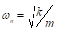, needs to be modified because the mass of the beam itself (mb = Wbeam/g), which is distributed over its height, is not negligible compared with the mass at the top (m = Wblock/g). The equivalent mass for the case of a cantilever beam is (m+0.23mb), where m is the mass at the top and mb is the distributed mass of the beam. The stiffness k is given by the reciprocal of the deformation () caused at the top of the cantilever by a unit force: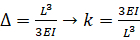(Eq. 11)

where L is the length of the beam, E is the modulus of elasticity, and I is the moment of inertia. I is given by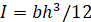, where b is the width and h is the thickness of the beam. Thus, the natural circular frequency of a cantilever beam, including its self-weight is: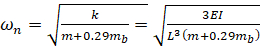(Eq.12)

Based on this equation, the predicted natural frequencies are calculated in the Table 1.

 Beam Number Length (in) Width (in.) Thick. (in.) I (in.4) E (ksi) Weight (lbs) Beam Weight (lbs.) Effective Mass (lbs-sec.2/in) Natural frequency (cycles per second) 1 12.0 1.002 0.124 1.59E-04 10200 0.147 0.149 4.70E-04 2.45 2 16.0 1.003 0.124 1.59E-04 10200 0.146 0.199 4.97E-04 1.55 3 20.0 1.002 0.125 1.63E-04 10200 0.146 0.251 5.28E-04 1.09 4 24.0 1.003 0.125 1.63E-04 10200 0.148 0.301 5.63E-04 0.80 5 28.0 1.001 0.125 1.63E-04 10200 0.144 0.350 5.82E-04 0.62 6 32.0 1.000 0.124 1.59E-04 10200 0.146 0.397 6.15E-04 0.49 7 36.0 1.002 0.126 1.67E-04 10200 0.147 0.455 6.52E-04 0.41 8 40.00 1.000 0.125 1.63E-04 10200 0.148 0.500 6.81E-04 0.34

Table 1: Natural frequencies of the cantilever beams tested.

The measured and the theoretical values of the normal frequency for our model systems are compared in the Table 2. The actual natural frequencies were computed by carefully displacing the cantilever beam by 1 inch and then looking at the displacement vs. time response. The comparison below are made in terms of periods (Td , in sec.) as these were determined from Td = u0-u1, as shown in Figure 2(b). This requires care and patience to obtain reliable results. The demonstrations shown were only meant to give an overall illustration of the system behavior.

 Beam Number Natural frequency (cycles per second) Predicted Period (sec.) Actual Period (sec.) Error (%) 1 2.45 2.56 2.65 -3.33% 2 1.55 4.06 4.23 -4.22% 3 1.09 5.78 6.79 -17.52% 4 0.80 7.84 8.04 -2.54% 5 0.62 10.06 10.63 -5.70% 6 0.49 12.79 13.04 -1.97% 7 0.41 15.32 16.78 -9.50% 8 0.34 18.59 20.56 -10.59%

Table 2. Comparison of results.

The differences stem primarily from the fact that the beams are not rigidly attached to the wooden base, and the added flexibility at the base increases the period of the structure. Another source of error is that the damping was not accounted for in the calculations, because damping is very difficult to measure and amplitude dependent.

Next, from each of the displacement vs. time histories, extract the maximum value for each frequency and plot the magnitude of the displacement vs. normalized frequency like that in Figure 3. An example is shown in Figure 4, where we have normalized frequency versus the first natural frequency (Beam Number 1) and plotted the maximum displacement of that beam when the shake table was subjected to a varying sinusoidal deformation with amplitude of 1 in.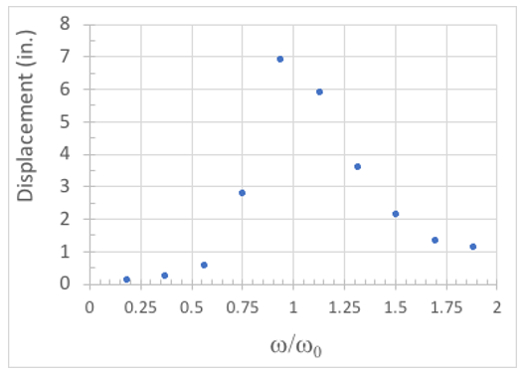Figure 4: Deformation of Beam #1 vs. normalized table frequency.

Initially, when the ratio of ω/ωn is small, there is not much response as the energy input from the table motion does not excite the model. As ω/ωn approaches 1, there is a very significant increase in the response, with the deformations becoming quite large. The maximum response is reached when ω/ωn is very close to 1. As the normalized frequency increases beyond ω/ωn = 1, the dynamic response begins to die down; when ω/ωn becomes large we are in a situation where the load is being applied very slowly with respect to the natural frequency of the structure, and the deformation should become equal to that from a statically applied load.

The intent of these experiments is primarily to show the changes in behavior qualitatively, as shown in the demonstrations for the two frame structures. Obtaining results similar to those in Figures 3 and 4 requires great care and patience as sources of friction and similar will affect the amount of damping and thus shift the curves similar to those in Figure 3(c) to the left or right as the actual damped frequency,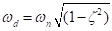, changes.

Applications and Summary

In this experiment, the natural frequency and damping of a simple cantilever system were measured by using shake tables. Although the frequency content of an earthquake is random and covers a large bandwidth of frequencies, frequency spectra can be developed by translating the acceleration time history into the frequency domain through the use of Fourier transforms. If the predominant frequencies of the ground motion match that of the structure, it is likely that the structure will undergo large displacement and consequently be exposed to great damage or even collapse. Seismic design looks at the acceleration levels expected form an earthquake at a given location based on historical records, distance to the earthquake source, the type and size of the earthquake source, and the attenuation of the surface and body waves to determine a reasonable level of acceleration to be used for design.

What the general public often does not realize is that current seismic design provisions are only intended to minimize the probability of collapse and loss of life in the case that a maximum credible earthquake occurs to an acceptable level (around 5% to 10% in most cases). While structural designs to obtain lower probabilities of failure are possible, they begin to become uneconomical. Minimizing losses and improving resilience after such an event are not explicitly considered today, although such considerations are becoming more common, as many times the contents of a building and its functionality may be much more important than its safety. Consider for example the case of a nuclear power plant (like Fukushima in the 2011 Great Kanto Earthquake), a residential ten-story building in Los Angeles, or a computer chip manufacturing facility in Silicon Valley and their exposure and vulnerability to seismic events.

In the case of the nuclear power plant, it may be desirable to design the structure to minimize any damage given that the consequence of even a minimal failure can have very dire consequences. In this case, we should try to locate this facility as far away as possible from earthquake sources to minimize exposure, because minimizing vulnerability to the desired level is very difficult and expensive. The reality is that it is prohibitively expensive to do this given the public's desire to avoid not only a Fukushima-type incident, but also even a more limited one, like the nuclear disaster on Three Mile Island.

For the multi-story building in Los Angeles, it is more difficult to minimize exposure because a large network of seismic faults with somewhat unknown return periods is nearby, including the San Andreas Fault. In this case, the emphasis should be on robust design and detailing to minimize the structure's vulnerability; the owners of the residences should be conscious that they are taking a significant risk should an earthquake occur. They should not expect the building to collapse, but the building may be a complete loss if the earthquake is of a large enough magnitude.

For the computer chip plant, the problems may be completely different because the structure itself may be quite flexible and outside the frequency range of the earthquake. Thus, the structure may not suffer any damage; however, its contents (chip manufacturing equipment) may be severely damaged, and chip production could be disrupted. Depending on the specific set of chips being manufactured at the facility, the economic damage both to the owner of the facility and to the industry as a whole can be tremendous.

These three examples illustrate why one needs to develop resilient design strategies for our infrastructure. To reach this goal we need to understand both the input (ground motion) and output (structural response). This issue can only be addressed through a combined analytical and experimental approach. The former is reflected in the equations listed above, while the latter can only be achieved through the experimental work done through quasi-static, pseudo-dynamic, and shake table approaches.X